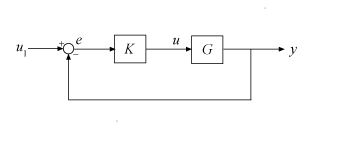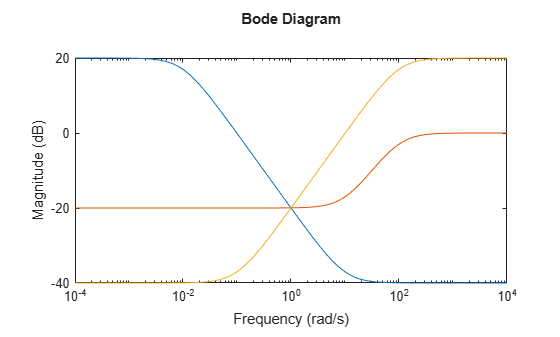# mixsyn

Mixed-sensitivity H synthesis method for robust control loop-shaping design

## Syntax

``[K,CL,gamma,info] = mixsyn(G,W1,W2,W3)``
``[K,CL,gamma] = mixsyn(G,W1,W2,W3,gamTry)``
``[K,CL,gamma] = mixsyn(G,W1,W2,W3,gamRange)``
``[K,CL,gamma] = mixsyn(___,opts)``
``[K,CL,gamma,info] = mixsyn(___)``

## Description

example

````[K,CL,gamma,info] = mixsyn(G,W1,W2,W3)` computes a controller that minimizes the H∞ norm of the weighted closed-loop transfer function $M\left(s\right)=\left[\begin{array}{c}{W}_{1}S\\ {W}_{2}KS\\ {W}_{3}T\end{array}\right],$where S = (I + GK)–1 and T = (I – S) is the complementary sensitivity of the following control system.You choose the weighting functions `W1,W2,W3` to shape the frequency responses for tracking and disturbance rejection, controller effort, and noise reduction and robustness, respectively. For details about how to choose weighting functions, see Mixed-Sensitivity Loop Shaping.`mixsyn` computes the controller `K` that yields the minimum ||M(s)||∞, which is returned as `gamma`. For the returned controller K,$\begin{array}{c}{‖S‖}_{\infty }\le \gamma |{W}_{1}^{-1}|\\ {‖KS‖}_{\infty }\le \gamma |{W}_{2}^{-1}|\\ {‖T‖}_{\infty }\le \gamma |{W}_{3}^{-1}|.\end{array}$```
````[K,CL,gamma] = mixsyn(G,W1,W2,W3,gamTry)` calculates a controller for the target performance level `gamTry`. Specifying `gamTry` can be useful when the optimal controller performance is better than you need for your application. In that case, a less-than-optimal controller can have smaller gains and be better conditioned numerically. When `W1,W2,W3` capture the desired limits on the gains of S, KS, and T, use `gamtry` = 1 to just enforce those limits. If `gamTry` is not achievable, `mixsyn` returns `[]` for `K` and `CL`, and `Inf` for `gamma`. ```
````[K,CL,gamma] = mixsyn(G,W1,W2,W3,gamRange)` searches the range `gamRange` for the best achievable performance. Specify the range with a vector of the form `[gmin,gmax]`. Limiting the search range can speed up computation by reducing the number of iterations performed by `mixsyn` to test different performance levels. ```
````[K,CL,gamma] = mixsyn(___,opts)` specifies additional computation options. To create `opts`, use `hinfsynOptions`. Specify `opts` after all other input arguments.```
````[K,CL,gamma,info] = mixsyn(___)` returns a structure containing additional information about the H∞ synthesis computation. You can use this argument with any of the previous syntaxes.```

## Examples

collapse all

Use `mixsyn` for sensitivity and complementary sensitivity loop shaping. Create a plant model and weighting functions that:

• Shape the sensitivity function for reference tracking and disturbance rejection (`W1 = 1/S` large inside the control bandwidth).

• Shape the complementary sensitivity for robustness and noise attenuation (`W3 = 1/T` large outside the control bandwidth).

• Limit the control effort (`W2 = 1/KS` large inside the control bandwidth).

```s = zpk('s'); G = (s-1)/(s+1)^2; W1 = makeweight(10,[1 0.1],0.01); W2 = makeweight(0.1,[32 0.32],1); W3 = makeweight(0.01,[1 0.1],10); bodemag(W1,W2,W3)```Design the controller.

`[K,CL,gamma] = mixsyn(G,W1,W2,W3);`

`mixsyn` shapes the singular values of the sensitivity function `S`, the complementary sensitivity function `T`, and the control effort `R = K*S`. Examine the results of the synthesis and the shapes of these transfer functions.

```S = feedback(1,G*K); KS = K*S; T = 1-S; sigma(S,'b',KS,'r',T,'g',gamma/W1,'b-.',ss(gamma/W2),'r-.',gamma/W3,'g-.',{1e-3,1e3}) legend('S','KS','T','GAM/W1','GAM/W2','GAM/W3','Location','SouthWest') grid```## Input Arguments

collapse all

Plant, specified as a dynamic system model such as a state-space (`ss`) model. `G` can be any LTI model. `mixsyn` assumes the following control structure.If `G` is a generalized state-space model with uncertain or tunable control design blocks, then `mixsyn` uses the nominal or current value of those elements.

Weighting functions, specified as dynamic system models. Choose the weighting functions `W1,W2,W3` to shape the frequency responses for tracking and disturbance rejection, controller effort, and noise reduction and robustness. Typically:

• For good reference-tracking and disturbance-rejection performance, choose `W1` large inside the control bandwidth to obtain small S.

• For robustness and noise attenuation, choose `W3` large outside the control bandwidth to obtain small T.

• To limit control effort in a particular frequency band, increase the magnitude of W2 in this frequency band to obtain small KS.

If one of the weights is not needed, set it to `[]`. For instance, if you do not want to restrict control effort, use `W2 = []`.

Use `makeweight` to create weighting functions with the desired gain profiles. For details about choosing weighting functions, see Mixed-Sensitivity Loop Shaping.

If `G` has NU inputs and NY outputs, then `W1,W2,W3` must be either SISO or square systems of size NY, NU, and NY, respectively.

Because S + T = I, `mixsyn` cannot make both S and T small (less than 0 dB) in the same frequency range. Therefore, when you specify weights for loop shaping, there must be a frequency band in which both `W1` and `W3` are below 0 dB.

Target performance level, specified as a positive scalar. `mixsyn` attempts to compute a controller such that the H of the weighted closed-loop system M(s) does not exceed `gamTry`. If this performance level is achievable, then the returned controller has `gamma``gamTry`. If `gamTry` is not achievable, `mixsyn` returns an empty controller.

Performance range for search, specified as a vector of the form `[gmin,gmax]`. The `mixsyn` command tests only performance levels within that range. It returns a controller with performance:

• `gamma``gmin`, when `gmin` is achievable.

• `gmin` < `gamma` < `gmax`, when `gmax` is achievable and but `gmin` is not.

• `gamma` = `Inf` when `gmax` is not achievable. In this case, `mixsyn` returns `[]` for `K` and `CL`.

If you know a range of feasible performance levels, specifying this range can speed up computation by reducing the number of iterations performed by `mixsyn` to test different performance levels.

Additional options for the computation, specified as an options object you create using `hinfsynOptions`.

Use `opts` to specify options for the underlying `hinfsyn` computation (see Algorithms). Available options include:

• Display algorithm progress at the command line.

• Turn off automatic scaling and regularization.

• Specify an optimization method.

For information about all options, see `hinfsynOptions`.

## Output Arguments

collapse all

Controller, returned as a state-space (`ss`) model object or `[]`.

If you supply `gamTry` or `gamRange` and the specified performance values are not achievable, then `K` = `[]`.

Augmented closed-loop transfer function, returned as a state-space (`ss`) model object or `[]`. The augmented closed-loop transfer function is given by

`$M\left(s\right)=\left[\begin{array}{c}{W}_{1}S\\ {W}_{2}KS\\ {W}_{3}T\end{array}\right],$`

where S = (I + GK)–1 and T = (IS) is the complementary sensitivity of the unweighted control system. See Mixed-Sensitivity Loop Shaping.

The returned performance level `gamma` is the H norm of `CL`.

If you supply `gamTry` or `gamRange` and the specified performance levels are not achievable, then `CL` = `[]`.

Controller performance, returned as a nonnegative scalar value or `Inf`. This value is the performance achieved using the returned controller `K`, and is the H norm of `CL` (see `hinfnorm`). If you do not provide performance levels to test using `gamTry` or `gamRange`, then `gamma` is the best achievable performance level.

If you provide `gamTry` or `gamRange`, then `gamma` is the actual performance level achieved by the controller computed for the best passing performance level that `hinfsyn` tries. If the specified performance levels are not achievable, then `gamma` = `Inf`.

Additional synthesis data, returned as a structure or `[]` (if the specified performance level is not achievable). info contains data about the underlying `hinfsyn` computation used by `mixsyn` to minimize the H norm of the closed-loop transfer function M(s). For details about the meaning of the fields of `info`, see the `info` output argument of `hinfsyn`.

## Algorithms

`mixsyn` uses your weighting functions to generate an augmented plant `P = augw(G,W1,W2,W3)`. It then invokes `hinfsyn` to find a controller that minimizes the H norm of the closed-loop transfer function M(s) = LFT(P,K). For details, see Mixed-Sensitivity Loop Shaping.

## Version History

Introduced before R2006a

expand all